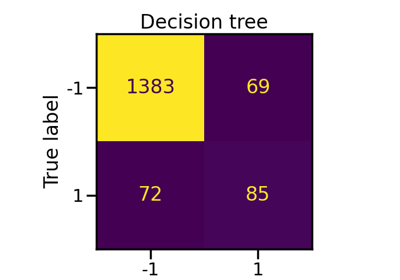# imblearn.ensemble.BalancedBaggingClassifier¶

class imblearn.ensemble.BalancedBaggingClassifier(base_estimator=None, n_estimators=10, *, max_samples=1.0, max_features=1.0, bootstrap=True, bootstrap_features=False, oob_score=False, warm_start=False, sampling_strategy='auto', replacement=False, n_jobs=None, random_state=None, verbose=0)[source]

A Bagging classifier with additional balancing.

This implementation of Bagging is similar to the scikit-learn implementation. It includes an additional step to balance the training set at fit time using a RandomUnderSampler.

Read more in the User Guide.

Parameters
base_estimatorobject, default=None

The base estimator to fit on random subsets of the dataset. If None, then the base estimator is a decision tree.

n_estimatorsint, default=10

The number of base estimators in the ensemble.

max_samplesint or float, default=1.0

The number of samples to draw from X to train each base estimator.

• If int, then draw max_samples samples.

• If float, then draw max_samples * X.shape samples.

max_featuresint or float, default=1.0

The number of features to draw from X to train each base estimator.

• If int, then draw max_features features.

• If float, then draw max_features * X.shape features.

bootstrapbool, default=True

Whether samples are drawn with replacement.

bootstrap_featuresbool, default=False

Whether features are drawn with replacement.

oob_scorebool, default=False

Whether to use out-of-bag samples to estimate the generalization error.

warm_startbool, default=False

When set to True, reuse the solution of the previous call to fit and add more estimators to the ensemble, otherwise, just fit a whole new ensemble.

sampling_strategyfloat, str, dict, callable, default=’auto’

Sampling information to sample the data set.

• When float, it corresponds to the desired ratio of the number of samples in the minority class over the number of samples in the majority class after resampling. Therefore, the ratio is expressed aswhereis the number of samples in the minority class andis the number of samples in the majority class after resampling.

Warning

float is only available for binary classification. An error is raised for multi-class classification.

• When str, specify the class targeted by the resampling. The number of samples in the different classes will be equalized. Possible choices are:

'majority': resample only the majority class;

'not minority': resample all classes but the minority class;

'not majority': resample all classes but the majority class;

'all': resample all classes;

'auto': equivalent to 'not minority'.

• When dict, the keys correspond to the targeted classes. The values correspond to the desired number of samples for each targeted class.

• When callable, function taking y and returns a dict. The keys correspond to the targeted classes. The values correspond to the desired number of samples for each class.

replacementbool, default=False

Whether or not to sample randomly with replacement or not.

n_jobsint, default=None

Number of CPU cores used during the cross-validation loop. None means 1 unless in a joblib.parallel_backend context. -1 means using all processors. See Glossary for more details.

random_stateint, RandomState instance, default=None

Control the randomization of the algorithm.

• If int, random_state is the seed used by the random number generator;

• If RandomState instance, random_state is the random number generator;

• If None, the random number generator is the RandomState instance used by np.random.

verboseint, default=0

Controls the verbosity of the building process.

BalancedRandomForestClassifier

Random forest applying random-under sampling to balance the different bootstraps.

EasyEnsembleClassifier

Ensemble of AdaBoost classifier trained on balanced bootstraps.

RUSBoostClassifier

AdaBoost classifier were each bootstrap is balanced using random-under sampling at each round of boosting.

Notes

This is possible to turn this classifier into a balanced random forest  by passing a sklearn.tree.DecisionTreeClassifier with max_features='auto' as a base estimator.

References

1

L. Breiman, “Pasting small votes for classification in large databases and on-line”, Machine Learning, 36(1), 85-103, 1999.

2

L. Breiman, “Bagging predictors”, Machine Learning, 24(2), 123-140, 1996.

3

T. Ho, “The random subspace method for constructing decision forests”, Pattern Analysis and Machine Intelligence, 20(8), 832-844, 1998.

4

G. Louppe and P. Geurts, “Ensembles on Random Patches”, Machine Learning and Knowledge Discovery in Databases, 346-361, 2012.

5

Chen, Chao, Andy Liaw, and Leo Breiman. “Using random forest to learn imbalanced data.” University of California, Berkeley 110, 2004.

Examples

>>> from collections import Counter
>>> from sklearn.datasets import make_classification
>>> from sklearn.model_selection import train_test_split
>>> from sklearn.metrics import confusion_matrix
>>> from imblearn.ensemble import BalancedBaggingClassifier
>>> X, y = make_classification(n_classes=2, class_sep=2,
... weights=[0.1, 0.9], n_informative=3, n_redundant=1, flip_y=0,
... n_features=20, n_clusters_per_class=1, n_samples=1000, random_state=10)
>>> print('Original dataset shape %s' % Counter(y))
Original dataset shape Counter({1: 900, 0: 100})
>>> X_train, X_test, y_train, y_test = train_test_split(X, y,
...                                                     random_state=0)
>>> bbc = BalancedBaggingClassifier(random_state=42)
>>> bbc.fit(X_train, y_train)
BalancedBaggingClassifier(...)
>>> y_pred = bbc.predict(X_test)
>>> print(confusion_matrix(y_test, y_pred))
[[ 23   0]
[  2 225]]

Attributes
base_estimator_estimator

The base estimator from which the ensemble is grown.

n_features_int

The number of features when fit is performed.

estimators_list of estimators

The collection of fitted base estimators.

estimators_samples_list of ndarray

The subset of drawn samples for each base estimator.

estimators_features_list of ndarray

The subset of drawn features for each base estimator.

classes_ndarray of shape (n_classes,)

The classes labels.

n_classes_int or list

The number of classes.

oob_score_float

Score of the training dataset obtained using an out-of-bag estimate.

oob_decision_function_ndarray of shape (n_samples, n_classes)

Decision function computed with out-of-bag estimate on the training set. If n_estimators is small it might be possible that a data point was never left out during the bootstrap. In this case, oob_decision_function_ might contain NaN.

__init__(self, base_estimator=None, n_estimators=10, *, max_samples=1.0, max_features=1.0, bootstrap=True, bootstrap_features=False, oob_score=False, warm_start=False, sampling_strategy='auto', replacement=False, n_jobs=None, random_state=None, verbose=0)[source]

Initialize self. See help(type(self)) for accurate signature.

## Examples using imblearn.ensemble.BalancedBaggingClassifier¶